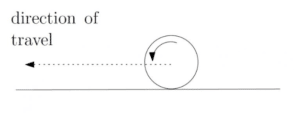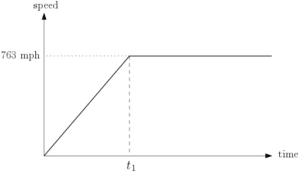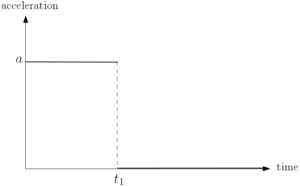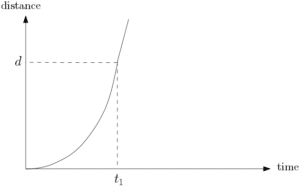# Motion in a Straight Line

Recall that acceleration at a given time measures the instantaneous change in velocity – see Quantities & Units in Mechanics. For motion in a straight line with constant acceleration, the speed along the line changes by the same amount every second. Hence, if speed is measured in metres per second (m/s) then acceleration is measured in metres per second per second (m/s/s). Note that when acceleration is constant, the average speed over a given distance or time is the mean of the initial and final speeds.Additionally, the distance moved in a straight line is average speed multiplied by time. For example, consider a ball rolling along a flat surface. If the ball rolls with speed 4 m/s and accelerates at a constant rate of 2 m/s/s, the ball will have speed 10 m/s after 3 seconds. The average speed over those 3 seconds is 7m/s. The distance moved is 21 metres.

It is important to note, however, that motion in a straight line may not have constant acceleration (see calculus below).

## Speed-Time GraphsWe can draw speed-time graphs for motion in a straight line. Consider a vehicle attempting to break the land speed record.The vehicle accelerates from rest to its maximum speed of 763 mph (miles per hour). At time t1 the vehicle ceases to accelerate and maintains its speed of 763 mph. This can be seen on a speed-time graph. Note that time is plotted on the x-axis and speed is plotted along the y-axis.The gradient on a speed-time graph gives you the instantaneous measure of acceleration. Note that acceleration is constant (a m/s/s) until time t1, then acceleration is 0.
Additionally, the total area beneath the speed-time graph gives you the total distance measured. In this example, the total distance is given by the area of the trapezium seen in the speed-time graph.The distance-time graph might look something like the graph on the right. If acceleration is constant, speed is linear and distance (displacement) will be quadratic (see calculus below).  If acceleration is 0, speed is constant and distance is linear. Consequently, the distance-time graph is linear beyond time t1.

Similarly to above, the gradient on a distance-time graph gives you the instantaneous measure of speed.

## Calculus of Position, Velocity and Acceleration

Velocity can be thought of as rate of change in position. Similarly, acceleration can be thought as rate of change in velocity. If position is given as a function of time, say r(t), velocity can be found be differentiating r(t). As a result, velocity is generally also a function of time. Analogously, acceleration a(t) can be found by differentiating velocity v(t). It follows that acceleration can be found by differentiating r(t) with respect to t twice. Mathematically, this can be written as

$v(t)=\frac{dr(t)}{dt},\hspace{20pt}a(t)=\frac{dv(t)}{dt}=\frac{d^2r(t)}{dt^2}$

Conversely, if velocity v(t) is given in terms of t,  integrating v(t) will give an expression for r(t). Similarly, if acceleration a(t) is given in terms of t, integrating a(t) will give v(t). It follows that r(t) can be found by integrating a(t) twice. Mathematically:

$r(t)=\int v(t)\, dt=\int\left(\int a(t)\, dt\right)dt,\hspace{10pt}v(t)=\int a(t)\, dt$

Note that when integrating, various integration constants may appear. Evidently, it is essential to use the information from the question to find the values of these integration constants.

## Motion in a Straight Line Examples

A ball rolls in a straight line with constant acceleration 4 m/s/s. Points P, Q and R lie on the line in that order. The ball has speed 9 m/s at the point Q. Additionally, the speed of the ball at R is 15 m/s. Given that the ball takes 1 second to get from P to Q, and treating the ball as a particle, find the distance P to R.

Recall that the SUVAT equation that relates displacement (S), initial velocity (U), time (T) and constant acceleration (A) is given by

$S=UT+\frac{1}{2}AT^2$

See SUVAT equations. For a particle with a constant acceleration of 4.7 m/s/s and an initial velocity of 7.05 m/s, use this equation to show that the final velocity of the particle is given by

$V=\frac{47}{20}(2T+3)$

A boomerang is thrown and is modelled as a particle moving along a straight line. The acceleration of the particle is modelled by the equation

$a(t)=-2t+2$

Given that the boomerang is thrown with an initial speed of 8 m/s, find the position of the boomerang when it turns back on itself.